top of page
Search

# Double sided counters

Updated: Oct 10, 2020

The models and images used in this blog are available to download at the end of the blog.

Double sided counters are nothing new to mathematics classrooms. These small plastic discs are versatile and can be used across the curriculum to unlock and deepen mathematical concepts. Here's how:

Double sided dexterous delights can represent and expose the structure of numbers from Early Years to Key Stage 3 and beyond. Using them with a place value grid allows focus on the value of each digit, understanding of the authority of the the position of digits in numbers and reading of numbers.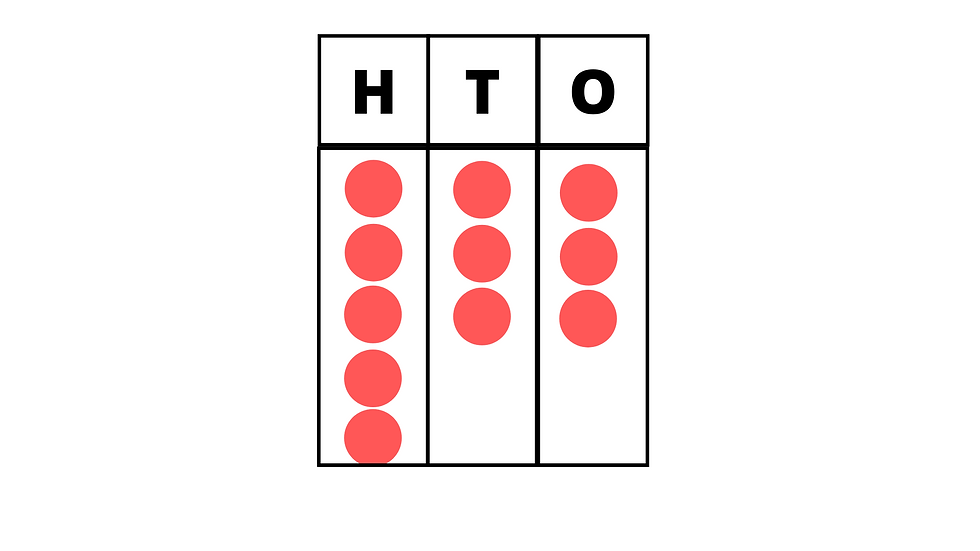One of the most simple ways can be using 5 frames and 10 frames. Children may show number bonds to 5 and 10 to show the depth of their understanding in key stage 1.

In key stage two, where keeping 5 and 10 frames may still be effective, counters may represent different values (such as one counter representing 5 or 10 etc). They may also be used to represent decimals, fractions and percentages. For this reason, they have strong relationships and effective links with addition and subtraction.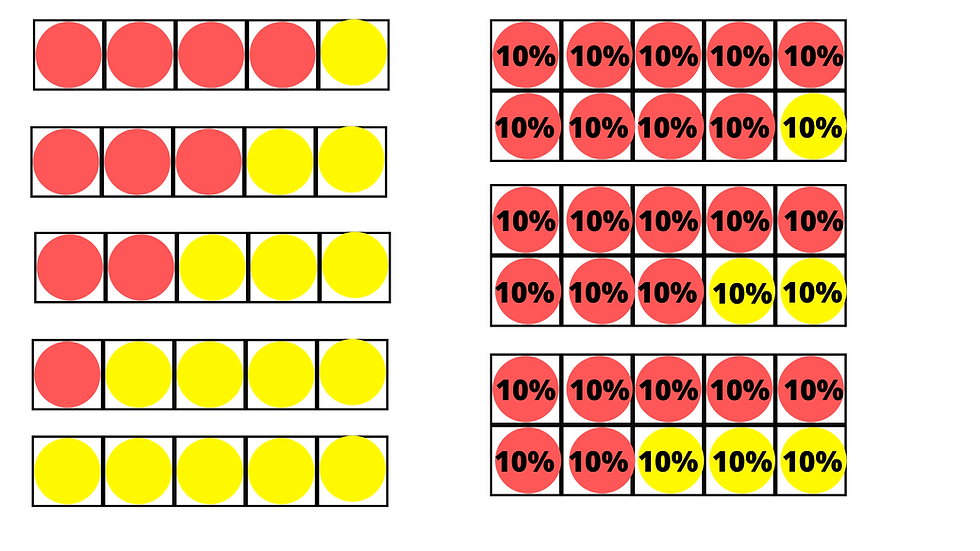Using them to introduce the concept of more than and less than can be a powerful way to introduce the concepts.

A number square and one double sided counter to obscure a number can be a powerful activity to develop reasoning and fluency around number. Finding one more, one less, ten more and/or ten less may be a good starting point for Key Stage one. Changing the language and questioning used can deepen the task for children at Key Stage two. Find the number that is ten more than ten more than 41, for example.

Introducing and strengthening understanding of inequality can be also achieved with double sided counters and lollipop sticks.The activities above use carefully chosen numbers and positions of the counters to allow the tasks to be opened up to asking "What is the same, what is different?".

We spoke earlier about using 10 frames, part-whole models, 100 squares and number tracks for addition and subtraction. But how might double sided counters be used in Key Stage two and beyond to support addition and subtraction?

Using double sided counters can anchor the gap between concrete, pictorial and abstract representations of mathematics. The images below suggest how this could be achieved as children progress to standard methods of addition and subtraction. Children would physically combine the counters for additions to calculate the sum and remove counters from the whole number for subtraction to leave the difference.

These also allow for the authority of the place value to be a focus to keep learning tight on the value of the digits.Multiplication and division.

Introducing multiplication as repeated addition is essential for understanding multiplication. Showing multiplication, including doubles and halves, can be a great way to start.This can then lead to arrays to represent multiplication. Having children draw the representation and then write the equation can deepen children's conceptual understanding for how multiplication works.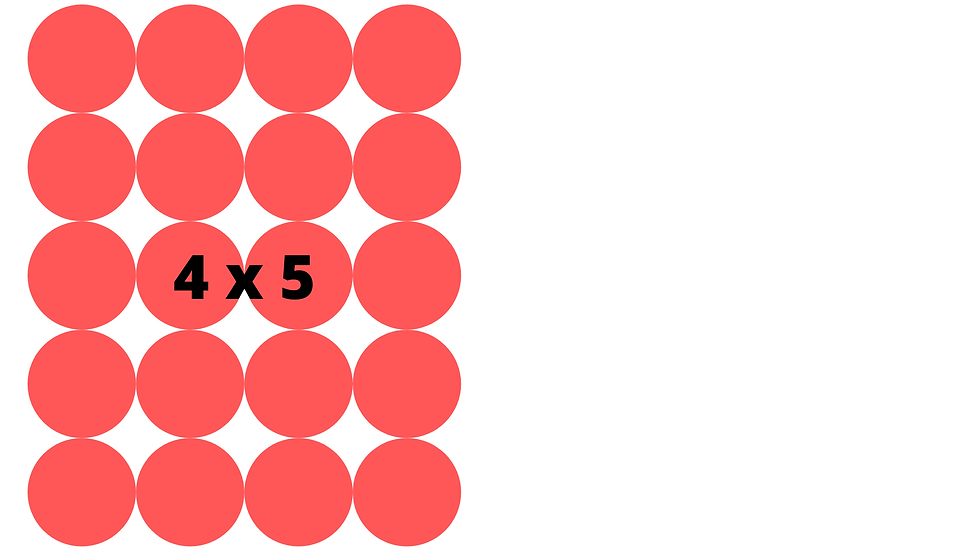The way children align and set out counters can prompt deep conceptual thinking as to the commutative nature of multiplication.Division by grouping can similarly be an effective way to share and divide a whole.Exploring patterns in lower Key Stage two can allow children to make connections between multiplication and division. This could use familiar resources such as 100 squares and number tracks. Obscuring numbers with counters can allow for meaningful reasoning. The examples below show:

• Multiples of 2 end in an even number.

• Multiples of two are always before or after an odd number.

• Multiples of 5 always end in 5 or 0.Similarly, number tracks can allow rich reasoning activities while gaining fluency of the ordinality of number. Using this with a counting stick is also a great way to practice counting across the mathematics curriculum: addition, subtraction, multiplication, division and counting in fractions.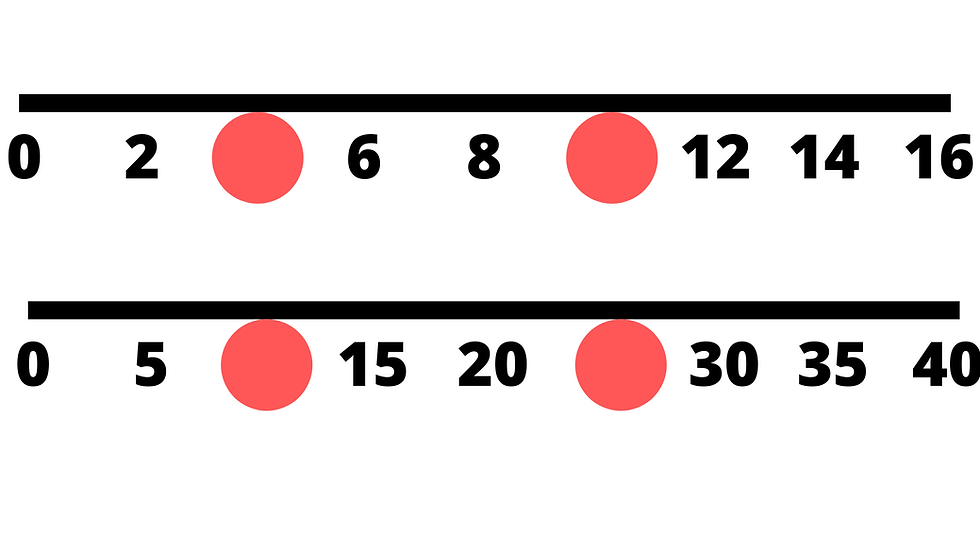Using counters in upper Key Stage two can also be effective when teaching multiplication and division:Fractions

Understanding fractions as part of a whole is an essential starting point in Year 2, when children start to learn about fractions. Exploring the whole and parts of the whole are essential starting points. This ought to be done in many different ways, expecting children to reason: "The whole is ___ equal parts, ___ is a part"

Similarly, children ought to familiarize themselves with counting with fractions, in the same way they do with whole numbers using number lines. Here, children will encounter whole and parts over time and develop fluency and ordinality (the position of the fraction in relation to others).The clear links to fractions of amounts can be explored using counters too.

As children progress to year lower Key Stage 2 and deepen their understanding of fractions as parts of wholes, children will begin to learn about equivalent fractions and simplifying fractions. Here, we show how counters can expose the structure of the fractions. The arrows show how counters may be moved and 'stacked' on top of other counters to reveal their equivalence and simplify the fractions.When multiplying fractions, using whiteboard pens to mark counters and arrange them into wholes and parts can be powerful for exploring addition (with same denominators) and multiplication.

Here we see 1/4 x 13 or (1/4 added 13 times) and the connections which can be made to expose improper fractions and mixed numbers.This image further strengthens the links between adding fractions and multiplying fractions (by a whole number).

Connecting fractional understanding to decimals and percentages is a powerful way of representing the structure of mathematics. With percent, children may see 100% as the whole, and 10% as a part. Giving a value to the whole allows then for linking into calculating percentages of amounts. Strengthening and deepening this concept allows for complex questions such as "If 30% of an amount = £15, what is 70% of the amount?". Adding a bar model can structure this clearly.

As we saw in Key Stage One, using a marker pen to show half of 10% = 5% may represent this concept clearly.Linking to decimals (and fractions) are powerful tools and may done in similar ways.Using a place value grid is already well documented, however, using whiteboard pens to show the structure of adding, subtracting, multiplying and dividing can be powerful. How they are organised is key to exposing the structure of mathematics. Adding a bar model can further reveal structures within an equation.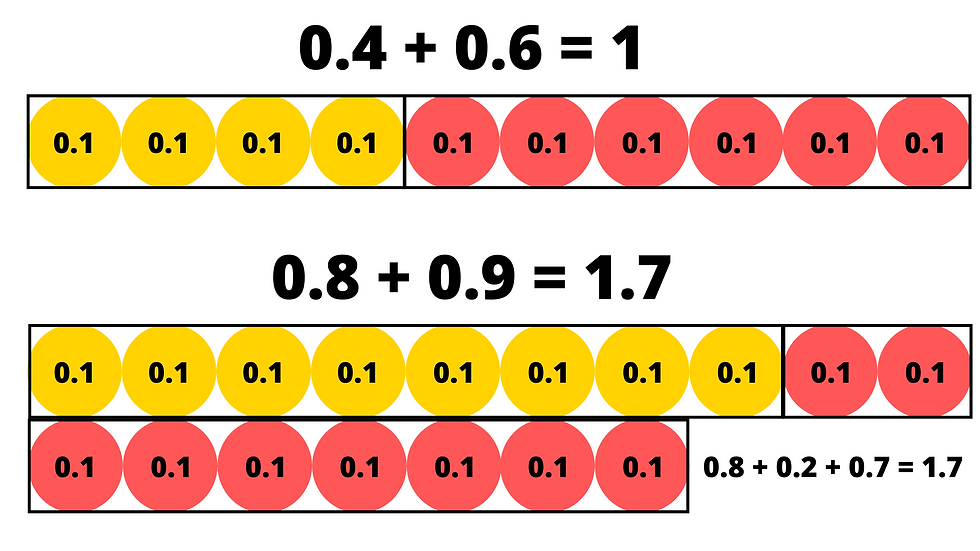Organising double sided counters into an array can allow connections between repeated addition, parts and wholes and strengthen to conceptual constructiveness of the mathematics. This image also shows 1/6 ÷ 4.Ratio and proportion

In a similar way as we explored with fractions, counters can be useful for ratio and proportion and exploring the simplest form.Some other uses may be useful for non-standard measurements, standard measures and/or telling time to an hour or half hour.Statistics

Pictograms and bar charts come to life when using double sided counters and allow rich discussions to develop into reasoning. Using them to distribute the mean average is also an effective strategy.I am sure you will agree, double sided counters are an excellent resource to introduce, strengthen and deepen conceptual understanding on mathematics.

Double sided counters
.pdf